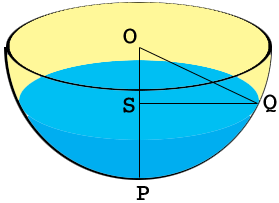SEARCH HOMEMath Central Quandaries & QueriesQuestion from Innocent: Abc is the section through a hemispherical bowl centre O radius 15cm. It contains water whose maximum depth is 8cm. What is the width of the water surface?Hi,

In my diagram $S$ and $P$ are on the surface of the water so $|SP| = 8$ cm, and $|OQ| = |OP| = 15$ cm.What is the value of $|OS|?$ Can you find $|SQ|?$ Maybe Pythagoras can help.

PennyMath Central is supported by the University of Regina and The Pacific Institute for the Mathematical Sciences.